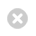Close

## UCL Mechanical Engineering Faculty of Engineering Sciences

Home# MECH0005 Introduction to Thermodynamics and Fluid Mechanics

## MECH0005 Introduction to Thermodynamics and Fluid Mechanics 1

FHEQ Level:
4 (Undergraduate Yr 1)
Term:
Term 2
UCL / ECTS Credits:
15 UCL / 7.5 ECTS
Previous Module Code:
MECH103P
Prerequisites:
MECH0003 Introduction to Mechanical Engineering

## Taught By

Dr Helen Czerski (Module Coordinator):
Helen Czerski
Dr Rama Balachandran:
Rama Balachandran

## Module Overview

This module provides an introduction to the broad concepts of thermodynamics and fluid mechanics, within a practical context which is relevant to industry. This is a necessary foundation for the engineers of the future. The particular aims of this module are:

1. To introduce and reinforce basic thermodynamic and fluid mechanics principles
2. To introduce the development of appropriate models which represent simplified practical systems
3. To enable students to demonstrate and articulate their understanding

The module consists of two thematic and overlapping areas: thermodynamics and fluid mechanics.  It will build on the introductory material covered in MECH0003 Introduction to Mechanical Engineering.

## Topics Covered

The topics that will be taught include:

Thermodynamics:

• Application of first law of thermodynamics
• Pure substance, real and perfect gases
• Second law of thermodynamics
• Reversibility
• Entropy
• Introduction to air standard gas power cycles, refrigeration and steam cycles

Fluid Mechanics:

• Importance of Fluid Mechanics
• Dimensional analysis: examples and power behind the methodology
• Control volume and system analysis, and the Reynolds Transport theorem
• Continuity equation, Momentum equation and energy equation
• Basic analysis of laminar flow

## Learning Outcomes:

Upon completion of this module students will be able to:

• Demonstrate knowledge and understanding of the essential facts, concepts, theories and principles underlying Thermodynamics and Fluid Mechanics.
• Appreciate the wider multidisciplinary context of the underlying theory, including its applications to engineering design and application to real world problems.
• Apply appropriate quantitative science, engineering and mathematical tools to the analysis of problems arising in Thermodynamics and Fluid Mechanics.

## Method of Instruction

This module is taught through:

• Lectures
• Workshops

## Assessment

This module is assessed entirely through an unseen written examination.

For more information about assessment please contact mecheng.ug-queries@ucl.ac.uk.